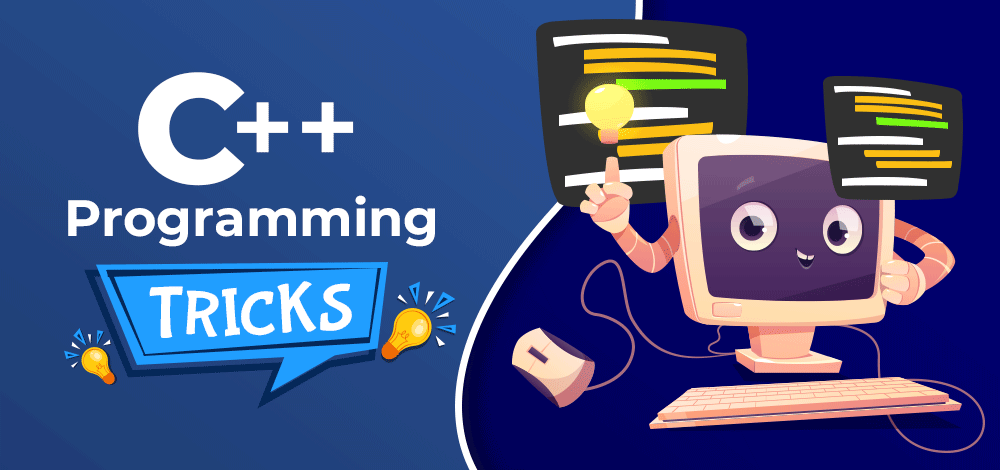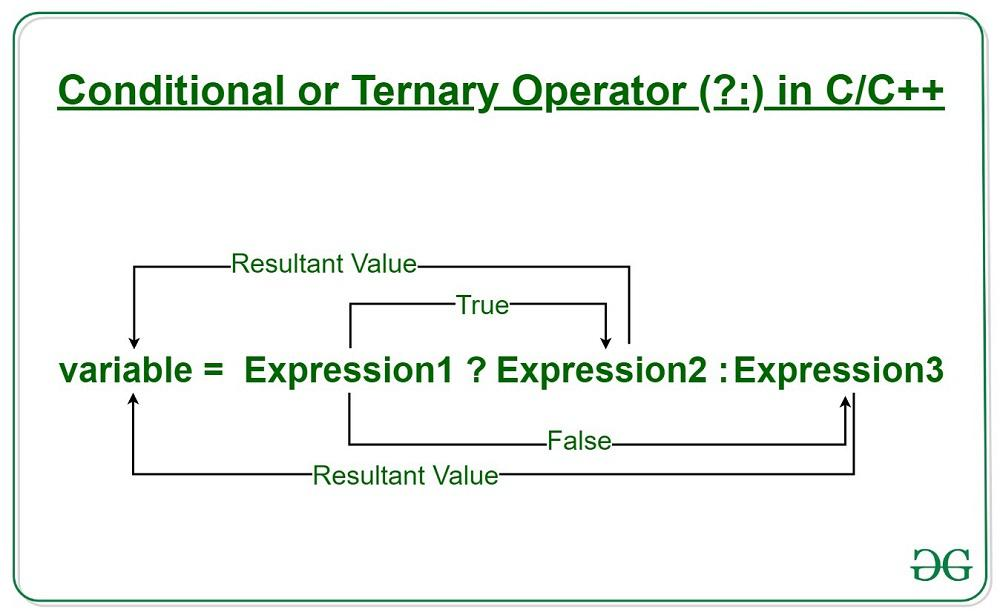Open in App
Not now

# 10 C++ Programming Tricks That You Should Know

• Difficulty Level : Hard
• Last Updated : 20 Jan, 2023

C++ Programming Language is a powerful, versatile, and compiled language, which means the source code is converted into machine language before the execution. In order to make your code as efficient and effective as possible, you should be aware of the following tricks and techniques. Hence, it’s better to know some C++ tricks and tips to reduce a few lines of code.In this article, you will come across some of the most important C++ tricks you should know in order to build interesting apps. These tips and tricks when applied help in saving time and reducing the code length. Let’s go through each of these tricks which help C++ developers to build amazing apps.

## Tricks for C++ Programming Language

So if you are curious to know about the 10 tricks of the C++ programming language then let’s get started.

### 1. Avoid Including Multiple Libraries

Generally, we include libraries at the start of the C++ code to perform certain operations as shown below.

Example:

## C++

 `#include ``#include ``#include ``#include ``using` `namespace` `std;` `int` `main()``{` `    ``cout << ``"GFG!"``;``    ``return` `0;``}`

`#include <iostream>`

This “iostream”  library performs the input-output operations in C++ code.

`#include<vector> `

The “vector” library allows us to perform operations on vectors.

`#include<set>`

Including this library, we can use operations on sets.

But, we have a better approach to replace these many libraries with just one library i.e, #include bits/stdc++.h> to include all standard libraries without adding them one at a time. It is especially useful in programming competitions where time is limited.

` #include<bits/stdc++.h>`

This includes all the standard libraries required in the program. So, we can avoid adding these many libraries separately to keep code as efficient and effective as possible.

### 2) Globally Defining Statements and Using them Locally Just by Some keywords

Yes, You can replace a statement just with a word by using the global definition in C++ Programming Language. For example, you have to print “GFG” at places in your program and you don’t want to write whole again and again; You can define a word with the same definition to avoid writing the same line multiple times.

`cout<<"GFG!"<<endl;`

Example 1:

## C++

 `#include ``using` `namespace` `std;``#define gfg cout << "GFG!"<<"\n";``int` `main() {` `    ``gfg``    ``gfg``    ``return` `0;``}`

Output:

``` GFG!
GFG! ```

Suppose, you’ve to convert all the integer data types into “long long”, so instead of changing each “int” into “long long”, we can simply define int with long long.

Example 2:

## C++

 `// C++ Program to demonstrate use``// of #define using long long``#include ` `using` `namespace` `std;` `#define int long long` `int32_t main()``{``    ``int` `x = 1e12 + 5;``    ``int` `y = 1e10;``    ``cout << x + y << endl;``}`

Output

`1010000000005`

This output as well as these x and y in the program are out of range of integer, but as we defined it earlier, it can now store long long.

Note: While defining ‘int’ as ‘long long’, we must always keep in mind that the main() function always returns the 32-bit integer, so we have to convert

int main (){ …} into  int32_t main(){ …}

### 3. Use Conditional/Ternary Operators

Although conditional operators follow the same algorithm as if-else statements, they take up less space and make it easier to write if-else statements in a shorter manner.

`b = ( a ? x : y  ) ==> if a is true then b = x else b = y`

In the above statement if a is true then b = x else b = y. It is similar to the below if-else statement.

```if(a)
{
b=x;
}
else
{
b=y;
}```Ternary operator

Example:

## C++

 `// C++ Program to Use Conditional/Ternary Operators``#include ``using` `namespace` `std;` `int` `main()``{` `    ``int` `x = 45;``    ``int` `y = 54;``    ``int` `z = (x > y) ? x : y;` `    ``cout << ``"Bigger of the two is "` `<< z << endl;``}`

Output

`Bigger of the two is 54`

### 4. Using STL (Standard Template Library)

The STL (Standard Template Library) is a collection of Algorithms, Containers, and functions. It’s very useful in programming and it saves so many lines of code just by replacing them with some small code snippet, defined in STL.

A) Algorithms: In this component, there are various algorithms for searching, and sorting.

For example, We can use binary search using STL.

Syntax:

`binary_search(startaddress, endaddress, valuetofind);`

Return type: It will return True if it finds the value, and False otherwise.

Time Complexity: O(log n)

We can use Sorting in a similar manner.

Syntax:

`sort(startaddress, endaddress); `

Time Complexity: O(n logn)

B) Containers:  Containers are used to store data. There are various types of containers that have unique properties. A few of them are as follows.

Vector:  A vector is a dynamic array. It can resize itself wherever required. Data is inserted in the end and can be popped out from the back itself. It is used where the data is dynamically passed.

Syntax:

`vector<dataType> vector_name;`

Stack: A stack is a container that works in LIFO (Last In First Out) principle. In stack, a new element is always inserted from the top and popped out from the end.

Syntax:

`stack<dataType> stack_name;`

Queue: Queue is a container that works in FIFO (First In First Out) principle. In stack, a new element is always inserted from the end (Back) and popped out from the front.

Syntax:

`queue<dataType> queue_name;`

Other important containers are Set, Multiset, Priority_queue, Deque, Map, Multimap, Unordered map, etc.

Thus by using STLs, not only do we reduce the lines of code but also we get more convenient data structures to work with. Hence It’s a good practice to use STLs.

### 5. Using Bitwise Operations

Bitwise operations are used when we have to operate on bits of a number. Since we operate on bits, it helps us operate on large numbers easily. Bitwise operations are very useful in programming. A few of the best use cases of Bitwise operations are as follows:

#### Checking odd numbers

We can check if a number is odd or even just by taking & ( bitwise and) of the number with 1.

```if(num & 1)
{
cout<<"Number is odd"<<endl;
}
else
{
cout<<"Number is even"<<endl;
}```

#### Using Left and Right Shift Operator

The left shift operator is used to multiply a number by 2 and the right shift operator is used to divide a number by 2 efficiently ( very useful in case of huge numbers).

Example:

## C++

 `// C++ Program to demonstrate use``// of Left shift and Right Shift``#include ``using` `namespace` `std;` `int` `main()``{` `    ``int` `x = 18;``    ``cout << ``"x << 1 = "` `<< (x << 1) << endl; ``// left shift``    ``cout << ``"x >> 1 = "` `<< (x >> 1) << endl; ``// right shift``    ``return` `0;``}`

Output

```x << 1 = 36
x >> 1 = 9```

#### Check if a number is a power of 2 or not.

Yes, this can be checked by using bitwise and operator. If a number is a power of 2, then it must have only one set bit in its binary representation. So,  if we subtract 1 from this number it’ll have all set bits except the one which was set previously. Hence, we take bitwise and of number and (number-1). If this value returns zero, then the number is a power of two, otherwise, it’s not.

Example:

## C++

 `// C++ program to check if a``// number is power of 2 or not``#include ``using` `namespace` `std;` `bool` `isPowerOfTwo(``int` `x) { ``return` `x && (!(x & (x - 1))); }` `// Driver code``int` `main()``{``    ``if` `(isPowerOfTwo(32))``        ``cout << ``"Yes\n"``;``    ``else``        ``cout << ``"No\n"``;``    ``if` `(isPowerOfTwo(31))``        ``cout << ``"Yes\n"``;``    ``else``        ``cout << ``"No\n"``;``    ``return` `0;``}`

Output

```Yes
No```

#### Swapping Two Variables without the third variable

The bitwise XOR operator can be used to swap two variables. The XOR of two numbers x and y returns a number that has all the bits as 1 wherever bits of x and y differ.

Example:

## C++

 `// C++ Program to swap two variables``// without the use of third variable``#include ``using` `namespace` `std;` `int` `main()``{` `    ``int` `x = 10;``    ``int` `y = 20;` `    ``cout << ``"Before Swap X: "` `<< x << ``" Y: "` `<< y << endl;` `    ``x ^= y;``    ``y ^= x;``    ``x ^= y;` `    ``cout << ``"After Swap X: "` `<< x << ``" Y: "` `<< y << endl;` `    ``return` `0;``}`

Output

```Before Swap X: 10 Y: 20
After Swap X: 20 Y: 10```

Time Complexity: O(1)
Auxiliary Space: O(1)

### 6. Using the ‘auto’ keyword to Drop Data Type

A) Instead of using int, string, or char data type, we can simply write auto for variable declaration.

Example:

```int a = 21;
char c = 'z';
string s= "GFG";
float x = 45.5;```

Can be declared as :

```auto  a = 21;
auto c = 'z';
auto s= "GFG";
auto x = 45.5;```

B) For traversing, instead of using for loop syntax we can use the ‘auto’ keyword.

Example:

```//Generally for loop is used as -
for(int i=0;i< v.size() ;i++)
{
//traversal
}

// But this can be simply written as-
for ( auto i: v){```

### 7. Finding the Number of Digits in a Number

In a positive number, if we have to find the number of digits, we can do it with the help of log(10) (Logarithm with base 10).

`Digit count of N = floor log10(number) `

Example:

## C++

 `// C++ Program to find the number of digits in a number``#include ``using` `namespace` `std;` `int` `Digit(``long` `long` `n) { ``return` `floor``(``log10``(n) + 1); }` `int` `main(``void``)``{``    ``long` `long` `n = 9878946457;``    ``cout << ``"Number of digits : "` `<< Digit(n);``    ``return` `0;``}`

Output

`Number of digits : 10`

### 8. Using the in-built GCD function

In many competitive programming problems, we get questions where we have to calculate the GCD (Greatest Common Divisor). So, it’s better to use the in-built function of GCD rather than writing Euclid’s theorem.

Syntax:

`__gcd(num1, num2);`

Returns: It returns 0 when both num1 and num2 are zero otherwise, it returns gcd of num1 & num2.

### 9. Use of “or” and “and”

When writing C++ code, “and” and “or” can help improve readability instead of & and |. Though this habit is not very productive but will help to use conditional operators easily.

Example:

## C++

 `// C++ Program to demonstrate use of or``#include ``using` `namespace` `std;` `int` `main()``{` `    ``int` `x = 10;``    ``if` `(x < 11 or x > 0) {``        ``cout << ``"YES"` `<< endl;``    ``}``    ``else` `{``        ``cout << ``"NO"` `<< endl;``    ``}``    ``return` `0;``}`

Output

`YES`

### 10. Using Pre-Increment Instead Post-Increment

Instead of using post-Increment, we should use pre-Increment as it is more efficient. Pre-Increment requires only one operation whereas post-Increment requires Two operations and keeps a copy of the previous operation. It is possible to use pre-increment both as an expression and as a statement, providing greater code flexibility.

`++i; // faster than post-increment`

These were some important tricks of the C++ programming language. One can follow these tricks to make their code clean, crisp, and short, and can save time in the contests by avoiding writing whole algorithms where it’s not needed.

## Conclusion

Programming in C++ is the most required skill that big tech companies look after. Now that you know about the most important C++ tricks used in programming, you can explore each and use them accordingly wherever required. These C++ tricks will help in reducing the number of lines in code and thus you can implement a fully-functional app with less complexity.

## Related Articles:

My Personal Notes arrow_drop_up